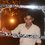# A doubt on Waves

Here is a question,

A string of mass $m$ and length $l$, both fixed at both ends is vibrating in its fundamental mode. The maximum amplitude is $a$ and the tension in the string is $T$. Find the energy of vibrations of the string in terms of $a$, $T$ and $l$.

This is how I answered,

Let the equation of wave be $y=f\left( x,t \right) =a\sin { \left( \omega t-kx \right) }$

Differentiating w.r.t $t$,

$\frac { \delta y }{ \delta t } ={ v }_{ p }=a\omega \cos { \left( \omega t-kx \right) }$

$\therefore$ Kinetic Energy, $K.E=\frac { 1 }{ 2 } m{ a }^{ 2 }{ \omega }^{ 2 }\cos ^{ 2 }{ \left( \omega t-kx \right) }$

$\therefore$Average Kinetic Energy, $\left< K.E \right> =\frac { \int _{ 0 }^{ T }{ \frac { 1 }{ 2 } m{ a }^{ 2 }{ \omega }^{ 2 }\cos ^{ 2 }{ \left( \omega t-kx \right) } dt } }{ \int _{ 0 }^{ T }{ dt } } =\frac { 1 }{ 4 } m{ a }^{ 2 }{ \omega }^{ 2 }$

Potential Energy, $P.E=\frac { 1 }{ 2 } m{ \omega }^{ 2 }{ a }^{ 2 }\sin ^{ 2 }{ \left( \omega t-kx \right) }$

$\therefore$ Average Potential Energy,$\left< P.E \right> =\frac { \int _{ 0 }^{ T }{ \frac { 1 }{ 2 } m{ a }^{ 2 }{ \omega }^{ 2 }\sin ^{ 2 }{ \left( \omega t-kx \right) } dt } }{ \int _{ 0 }^{ T }{ dt } } =\frac { 1 }{ 4 } m{ a }^{ 2 }{ \omega }^{ 2 }$

Therefore Total energy=$\left< K.E \right>+\left< P.E \right>=\frac { 1 }{ 2 } m{ a }^{ 2 }{ \omega }^{ 2 }$

Now,

${ \omega }^{ 2 }={ v }^{ 2 }{ k }^{ 2 }={ v }^{ 2 }\frac { 4{ \pi }^{ 2 } }{ { \lambda }^{ 2 } } =\frac { T }{ \frac { m }{ L } } \frac { 4{ \pi }^{ 2 } }{ { \lambda }^{ 2 } } =\frac { 2T{ \pi }^{ 2 } }{ m\lambda } =\frac { T{ \pi }^{ 2 } }{ mL }$

$\therefore$ Total Energy$=\boxed{\frac { T{ a }^{ 2 }{ \pi }^{ 2 } }{ 2L } }$

But the given answer is $\boxed{\frac { T{ a }^{ 2 }{ \pi }^{ 2 } }{ 4L} }$

Can someone explain this?Note by Anandhu Raj
5 years, 2 months ago

This discussion board is a place to discuss our Daily Challenges and the math and science related to those challenges. Explanations are more than just a solution — they should explain the steps and thinking strategies that you used to obtain the solution. Comments should further the discussion of math and science.

When posting on Brilliant:

• Use the emojis to react to an explanation, whether you're congratulating a job well done , or just really confused .
• Ask specific questions about the challenge or the steps in somebody's explanation. Well-posed questions can add a lot to the discussion, but posting "I don't understand!" doesn't help anyone.
• Try to contribute something new to the discussion, whether it is an extension, generalization or other idea related to the challenge.
• Stay on topic — we're all here to learn more about math and science, not to hear about your favorite get-rich-quick scheme or current world events.

MarkdownAppears as
*italics* or _italics_ italics
**bold** or __bold__ bold
- bulleted- list
• bulleted
• list
1. numbered2. list
1. numbered
2. list
Note: you must add a full line of space before and after lists for them to show up correctly
paragraph 1paragraph 2

paragraph 1

paragraph 2

[example link](https://brilliant.org)example link
> This is a quote
This is a quote
    # I indented these lines
# 4 spaces, and now they show
# up as a code block.

print "hello world"
# I indented these lines
# 4 spaces, and now they show
# up as a code block.

print "hello world"
MathAppears as
Remember to wrap math in $$ ... $$ or $ ... $ to ensure proper formatting.
2 \times 3 $2 \times 3$
2^{34} $2^{34}$
a_{i-1} $a_{i-1}$
\frac{2}{3} $\frac{2}{3}$
\sqrt{2} $\sqrt{2}$
\sum_{i=1}^3 $\sum_{i=1}^3$
\sin \theta $\sin \theta$
\boxed{123} $\boxed{123}$

## Comments

Sort by:

Top Newest

I think it will be a standing wave and not a progressive wave, whose equation you have written. Hence, amplitude for every point of the string is different.

- 2 years, 4 months ago

Log in to reply

I think you're right.
See this.
Unless, they meant only the energy due to the motion, which is the kinetic energy.

- 5 years, 2 months ago

Log in to reply

Again you are there to help me! Thank you, Sir :)

- 5 years, 2 months ago

Log in to reply

Are vah yyaar maja hi aa Gaya shandaar

- 3 years, 4 months ago

Log in to reply

×

Problem Loading...

Note Loading...

Set Loading...